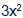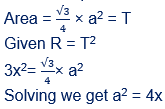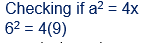# The sum of the perimeters of an equilateral - Quantitative Aptitude - CAT Geometry

## CAT 2020 - Slot 2 - Quantitative Aptitude - Geometry - Question 16 - The sum of the perimeters of an equilateral

Q. 16: The sum of perimeters of an equilateral triangle and a rectangle is 90 cm. The area, T, of the triangle and the area , R, of the rectangle, both in sq cm, satisfy the relationship R = T2. If the sides of the rectangle are in the ratio 1 : 3, then the length, in cm, of the longer side of the rectangle, is

##### 1. 272. 183. 214. 24

Sides of the rectangle are in the ratio 1 : 3

Take as x and 3x

Area of Rectangle == R

Take an equilateral triangle with side aNow from the options longer side 3x = 27

x = 9. Sides of the rectangle are 9 and 27.

If x = 9, Then a = 6### Past Year Question Paper & SolutionsCounselling Session
By IIM Mentor

#### Free Material Area